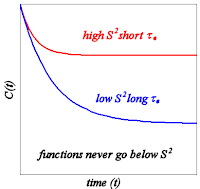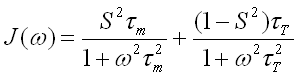I’ve seen a lot of really interesting articles in the past several days, though as always I admit that many things I find fascinating are sleep aids to others. I was going to write about the most esoteric article first, but as I started writing out an explanation I realized I needed to split things into two posts. The next post will be about an article I read last week in JACS ASAP pre-publication. But this post is about an article that appeared in JACS when I was about four years old. In it, Giovanni Lipari and Attila Szabo introduced a way of describing the dynamic information obtained from NMR so useful and successful that it has not been supplanted even after a quarter of a century. In addition, the article is really wonderfully written, and if you are a professional then I highly recommend reading it (the citation is at the bottom of this post). If you are not a professional, then read this post instead.

Regrettably, it is impossible to talk about this without using mathematics, but I promise you will not be forced to endure anything taxing, just exponents and multiplication. The Lipari-Szabo model-free formalism derives much of its power from its relative simplicity, so I promise this will not be too painful, and for those of my family who are interested, you will learn what all that junk I talk about means. Practicing NMR spectroscopists will notice a few differences in terminology and some (hopefully slight) conceptual fudging in what follows, but hey, you should be reading the original article anyway.So. Let’s imagine we have a really simple chemical system, like a single bond between two atoms, which we will call A and X (see right). Now, unless our AX compound is very, very cold it will be moving around all the time. Exactly how fast it moves will depend on what is around it (AX moves quicker through water than through honey) and what temperature it is (AX moves faster when warm), but unless we are at absolute zero, it will move. We can imagine two kinds of motion. AX can move forward or sideways: this is called translational motion, and having mentioned it, we won’t worry about it anymore. In fact, we will temporarily pretend that it does not even happen. Also, AX can tumble—for instance, it could rotate around some point in the center of the bond, or swing around like a clock hand, or wobble at the ends (as shown). This is the motion we will consider.Suppose you had a system like this and you wanted to come up with a way to show how it was moving. Well, one thing you could do is draw a picture or make a movie showing how quickly it tumbled. However, this would be a pretty awkward way to convey the data and might not be very informative. It might be better to try to come up with a single number that tells us how much AX has tumbled. Well, one way to do that would be to monitor AX over time, starting at some random time i, and compare the bond vector at any time i+t to the bond vector at time i. We could assign to any time t a number representing how likely it is to find AX in the same orientation at t that it had at i. Obviously, early on, this probability would be very high, and the larger t got, the smaller the probability would get. An example graph is over there on the left. This is called a correlation function, because it represents the correlation between the AX orientation at time i and the orientation at time i+t.

Obviously, for any real system the correlation function will be bumpy and difficult to represent mathematically. However, it turns out that we can approximate a correlation function reasonably well using an exponential decay, that is, a function based on a negative exponent of Euler’s number. Thus we have:where C(t) is the correlation function, t is the time (our x axis), and τm is a time constant, the value of which will depend on the speed with which AX tumbles. And now we have a single number that tells us about the tumbling. This number, τm, expresses the speed at which AX tumbles, and is called the rotational correlation time of the molecule. This particular number has been given an ungodly different number of subscripts, but I will use the original notation of Lipari and Szabo and call it τm—this means it is the molecular correlation time.

Well, so far so good, but a piddly system that just consists of two atoms isn’t really that interesting. Your typical masochistic biomolecular NMR researcher is after molecules (proteins) that have hundreds or thousands of atoms, and even more bond vectors. Now, the picture would still be very simple if these very large molecules were perfectly rigid, but in fact proteins are very flexible and each bond vector is capable of moving on its own, independent of the overall molecular motion. So if we imagine that AX is part of a protein (so that X is bonded to other atoms and A is free) we have to figure out how to represent two motions. This might seem like a major problem, but we still have some hope.First of all, let’s pretend for a moment that the protein doesn’t tumble. Well, in that case we could just use the same trick we did earlier, and draw up a correlation function to describe how AX moves inside the protein. Now, we have one difference from the earlier case. Because AX is now part of a much larger system, the correlation function does not decay to zero. Instead, it will decay to some static value, depending on how tightly constrained the bond motion is. If the bond is absolutely rigid, then that value is 1, meaning you have a 100% chance of finding the bond in the same position no matter when you look. If AX is completely flexible, then the ultimate value is 0. So, we’ll call this number, which can take values from 0 to 1 and describes the rigidity of the bond, the generalized order parameter and denote it with S2. Now, aside from the little wrinkle we just introduced, this is still an exponential decay. So, we need a correlation time, which we will denote with τe (an “extra” correlation time). So now we have a second correlation function:We’ll call our first function CM(t) (for molecular motion) and the second one CE(t) (for the extra motion). Obviously, these two correlation functions together will completely describe the motion of the bond. But how do we stick them together? If the two motions are correlated (i.e. the probabilities are not independent) this is really quite difficult to do in any general way. But, if the extra motion (the bond moving on its own) really is completely independent of the molecular motion (tumbling), then we can just multiply the functions to get CT(t), the total correlation function:whereSo now we have an expression to describe the correlation function (i.e. the motion) of the bond, in terms of three parameters (τT is just a combination of two others so it doesn’t count):

τm – the MOLECULAR correlation time
S2 – the ORDER PARAMETER
τe – the EXTRA correlation time

That’s great, but if we could actually measure the correlation function directly we’d hardly need all these parameters, right? We could just look at the correlation functions and compare them. But, it turns out that it is extremely difficult to measure the correlation function of a bond like this directly. Using NMR, however, we can measure something that is related, called the spectral density and denoted by J(ω). What the spectral density is and how NMR can be used to measure it is something for another time (this post is already really freakin’ long). The upshot of all of it is, though, that our model of the correlation function CT(t) translates into this model of the spectral density:This should be familiar to NMR spectroscopists, though I have omitted scaling factors for clarity. S2, τm, and τT mean exactly the same thing they did in equations 3 and 4 above. The ω represents a frequency, which should give you a little hint about what’s going on here: the spectral density is the correlation function represented in terms of frequency, rather than time. This information can be extracted from various relaxation rates measured in NMR spectrometers.

So, now we have a lovely little model of bond motion and a way to get at the parameters experimentally. But it isn’t really a model, because we haven’t made any sort of assumptions about the nature of the movement involved. We’ve merely stipulated that there is a global motion, that there is an internal motion, and that these motions are independent. Hence this is called a model-free formalism for describing dynamics. The Lipari-Szabo model-free formalism is extremely powerful because it is very general—the main advantage of this approach is that it can be used to describe almost any motion of a bond. But, the main disadvantage of this approach is that it can be used to describe almost any motion of a bond. Using S2, τm, and τe you can only find out how much a bond is moving, and how fast. The direction and distribution of motions is unknown. So if you want to know, for instance, whether two bonds move in a synchronous or asynchronous manner, the model-free approach can’t help you.

Unless you have extremely good luck, you need computer simulations of protein motions to get those kind of answers. All-atom molecular dynamics simulations of proteins have the advantage of explicitly telling you exactly where every bond is moving at every moment. They have some disadvantages too, but the major one of importance for this discussion is that they have historically been very bad at reproducing the dynamics information that comes from NMR, especially when it comes to the motions of side chains. The resolution of that problem will be the subject of my next post.

Lipari, G. and Szabo, A. “Model-free approach to the interpretation of nuclear magnetic resonance relaxation in macromolecules. 1. Theory and range of validity” J. Am. Chem. Soc. 1982: 104(17); pp. 4546-4559.

### 5 Responses to “The model-free dynamics formalism of Lipari and Szabo”

1.Wow… you made it clear and simple. This was very helpful to me.=) Thanks!

And how about Fourier transform? Do you have any simple explanation for it?

2.Your explanation was incredible, complete, easy and brief. Though I was expecting some help with all that thing of chosing a model considering the anisotropy of the diffusion tensor… I mean like for example, when you use Palmer's Modelfree or Blackledge's Tensor… I wonder if I could contact you via email to discuss about it ?

3.This is a great summary of a complex paper….don't have to read the article now which I wouldn't have understood well anyway.

4.Hey sparky, It was impressive!!! I just came through google to your blog..

5.Hello Michael.
Thank you very much for the Lipari-
Szabo model free formalism post. I have tried to understand this at school but I was unable to until now.
Michael, please post about the spectral density and how it can be obtained via NMR. You mentioned that relaxation rates are involved. Why is this? How can relaxation rates reveal the order parameter and correlation times? Also, Michael, please consider sending me a comment to help me to understand the difference between C(t) and the order parameter S^2; it seems to be that they mean the same thing, except that S^2 only goes from 0 to 1 while C(t) might go above 1 ?
Thank you again very much.

glen

Sorry, the comment form is closed at this time.